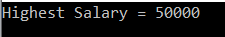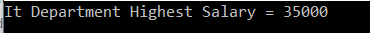# Linq Max in C#

## Linq Max in C# with Examples

In this article, I am going to discuss the Linq Max in C# with examples. Please read our previous article before proceeding to this article where we discussed the Sum Aggregate Functions in C# with some examples. As part of this article, we are going to discuss the following pointers.

1. What is Linq Max in C#?
2. Multiple examples using both Method and Query syntax.
##### What is Linq Max in C#?

The Linq Max in C# is used to returns the largest numeric value from the collection on which it is applied. Let us understand the Max() method with some examples.

##### Example1:

The following example returns the largest number from the collection.

```using System;
using System.Linq;
namespace LINQDemo
{
class Program
{
static void Main(string[] args)
{
int[] intNumbers = new int[] { 10, 80, 50, 90, 60, 30, 70, 40, 20, 100 };

//Using Method Syntax
int MSLergestNumber = intNumbers.Max();

//Using Query Syntax
int QSLergestNumber = (from num in intNumbers
select num).Max();

Console.WriteLine("Largest Number = " + MSLergestNumber);

}
}
}
```

Once you run the application, it will display the output as 100.

Note: We don’t have any operator called Max in Linq query syntax. So here we need to use the mixed syntax.

##### Example2: Linq Max with filter in C#

Now we need to return the largest number from the collection where the number is less than 50.

```using System;
using System.Linq;
namespace LINQDemo
{
class Program
{
static void Main(string[] args)
{
int[] intNumbers = new int[] { 10, 80, 50, 90, 60, 30, 70, 40, 20, 100 };

//Using Method Syntax
int MSLergestNumber = intNumbers.Where(num => num < 50).Max();

//Using Query Syntax
int QSLergestNumber = (from num in intNumbers
where num < 50
select num).Max();

Console.WriteLine("Largest Number = " + MSLergestNumber);

}
}
}```

It will print the output as 40.

##### Example3: Linq Max with Predicate

Instead of using the where method to filter the data, you can also use the other overloaded version of the Max method which takes a Predicate and within that predicate, you can write the logic to filter the data as shown in the below example.

```using System;
using System.Linq;
namespace LINQDemo
{
class Program
{
static void Main(string[] args)
{
int[] intNumbers = new int[] { 10, 80, 50, 90, 60, 30, 70, 40, 20, 100 };

//Using Method Syntax
int MSLergestNumber = intNumbers.Max(num => {
if (num < 50)
return num;
else
return 0;
});

Console.WriteLine("Largest Number = " + MSLergestNumber);

}
}
}```

It should give the same output as the previous example.

##### Max Method Working with Complex Type in C#:

We are going to work with the following Employee class.

```using System.Collections.Generic;
namespace LINQDemo
{
public class Employee
{
public int ID { get; set; }
public string Name { get; set; }
public int Salary { get; set; }
public string Department { get; set; }

public static List<Employee> GetAllEmployees()
{
List<Employee> listStudents = new List<Employee>()
{
new Employee{ID= 101,Name = "Preety", Salary = 10000, Department = "IT"},
new Employee{ID= 102,Name = "Priyanka", Salary = 15000, Department = "Sales"},
new Employee{ID= 103,Name = "James", Salary = 50000, Department = "Sales"},
new Employee{ID= 104,Name = "Hina", Salary = 20000, Department = "IT"},
new Employee{ID= 105,Name = "Anurag", Salary = 30000, Department = "IT"},
new Employee{ID= 106,Name = "Sara", Salary = 25000, Department = "IT"},
new Employee{ID= 107,Name = "Pranaya", Salary = 35000, Department = "IT"},
new Employee{ID= 108,Name = "Manoj", Salary = 11000, Department = "Sales"},
new Employee{ID= 109,Name = "Sam", Salary = 45000, Department = "Sales"},
new Employee{ID= 110,Name = "Saurav", Salary = 25000, Department = "Sales"}
};

return listStudents;
}
}
}
```

In the above Employee class, we define four properties such as ID, Name, Salary, and Department along with the GetAllEmployees() which will return the list of all the employees.

##### Example4:

The following example returns the highest salary among all the employees.

```using System;
using System.Linq;
namespace LINQDemo
{
class Program
{
static void Main(string[] args)
{
//Using Method Syntax
var MSHighestSalary = Employee.GetAllEmployees()
.Max(emp => emp.Salary);

//Using Query Syntax
var QSHighestSalary = (from emp in Employee.GetAllEmployees()
select emp).Max(e => e.Salary);

Console.WriteLine("Highest Salary = " + QSHighestSalary);

}
}
}
```

Output:##### Example5:

The following example returns the Highest Salary of IT department.

```using System;
using System.Linq;
namespace LINQDemo
{
class Program
{
static void Main(string[] args)
{
//Using Method Syntax
var MSHighestSalary = Employee.GetAllEmployees()
.Where(emp => emp.Department == "IT")
.Max(emp => emp.Salary);

//Using Query Syntax
var QSHighestSalary = (from emp in Employee.GetAllEmployees()
where emp.Department == "IT"
select emp).Max(e => e.Salary);

Console.WriteLine("It Department Highest Salary = " + QSHighestSalary);

}
}
}```

Output:##### Example6:

Let’s rewrite the previous example using a custom predicate.

```using System;
using System.Linq;
namespace LINQDemo
{
class Program
{
static void Main(string[] args)
{
//Using Method Syntax
var MSHighestSalary = Employee.GetAllEmployees()
.Max(emp => {
if (emp.Department == "IT")
return emp.Salary;
else
return 0;
});

Console.WriteLine("It Department Highest Salary = " + MSHighestSalary);